# Correlation

duality

A bijective mapping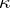between projective spaces of the same finite dimension such that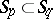implies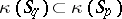. The image of a sum of subspaces under a correlation is the intersection of their images and, conversely, the image of an intersection is the sum of the images. In particular, the image of a point is a hyperplane and vice versa. A necessary and sufficient condition for the existence of a correlation of a projective space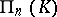over a division ringonto a space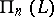over a division ringis that there exists an anti-isomorphism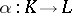, i.e. a bijective mapping for which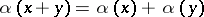,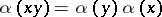; in that caseis dual to. Examples of spaces with an auto-correlation, i.e. a correlation onto itself, are the real projective spaces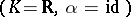, the complex projective spaces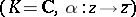and the quaternion projective spaces.

A polarity is an auto-correlationsatisfying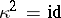. A projective spaceover a division ringadmits a polarity if and only ifadmits an involutory anti-automorphism, i.e. an anti-automorphismwith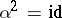.

A subspaceis called a null subspace relative to an auto-correlationif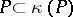for any point, and strictly isotropic if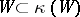. Any strictly isotropic subspace is a null subspace. A polarity relative to which the whole space is a null space is called a null (or symplectic) polarity (see also Polarity).

Let the projective spaceover a division ringbe interpreted as the set of linear subspaces of the (left) linear space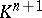over. A semi-bilinear form onis a mapping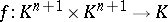together with an anti-automorphismofsuch that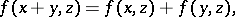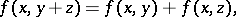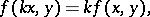In particular, ifis a field and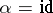, thenis a bilinear form. A semi-bilinear formis called non-degenerate providedfor all(all) implies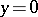(, respectively). Any auto-correlationofcan be represented with the aid of a non-degenerate semi-bilinear formin the following way: for a subspaceofits image is the orthogonal complement ofwith respect to: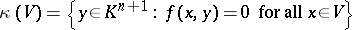(the Birkhoff–von Neumann theorem, ).is a polarity if and only ifis reflexive, i.e. ifimplies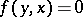. By multiplyingby a suitable element ofone can bring any reflexive non-degenerate semi-bilinear formand the corresponding automorphismin either of the following two forms:

1)is an involution, i.e., andIn this case one callssymmetric if(and hence necessarilyis a field) and Hermitian if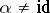.

2)(and henceis a field) andSuch anis called anti-symmetric.

A special example of a correlation is the following. Letbe a projective space over a division ring. Define the opposite division ring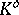as the set of elements ofwith the same addition but with multiplication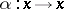is an anti-isomorphism fromontowhich defines the canonical correlation fromonto. The (left) projective space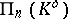, which can be identified with the right projective space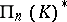, i.e. with the set of linear subspaces of the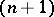-dimensional right vector space, is the (canonical) dual space of(cf. Projective algebra, the construction of).